# Music genre classification¶

## Problem¶

Categorizing music files according to their genre is a challenging task in the area of music information retrieval (MIR). We want to build a model which is able to perform this task and than explore ways to explain the models decisions especially for music files which do not easily fit into one single genre.

## Dataset¶

The dataset used is FMA: A Dataset For Music Analysis. https://github.com/mdeff/fma

Both preprocessed features like spectral_centroid, spectral_bandwidth and spectral_rolloff and raw audio files are available with this dataset, which is very handy.

## SVM¶

First simple SVM on the preprocessed features was used to have some baseline model. With this model accuracy 0.46375 was achieved, this is most likely a result of very careful feature enginneering, which was already done by the dataset creators.

More info and metrics like confusion matrix, recall, precision and f1-score, can be found in the notebook with the model itself.

## Preprocessing¶

The raw audio files had to be processed with librosa library - they were converted to mel spectrograms and saved as npz files. Those files are still pretty huge, so they are saved on google drive, not in this repo.

As I did not want to go trough the trouble of setting up tensorflow environment which i wanted use to power keras, I went with Google Colaboratory which is an online jupyter notebook environment with free GPU attached.

Problem I had to solve was related to the size of the dataset which is not small, fortunately there is a python package which allowed me to mount google drive as and read the data from there.

## CRNN¶

Convolutional Neural Networks (CNNs) are commonly used in image recognition related tasks. They perform convolution operation instead of matrix multiplication and are typically used in the early layers for understanding the 2D layout of the data. On the other hand RNNs excel in understand sequential data by making the hidden state at time t dependent on hidden state at time t-1. The neural network that we have built here use 1D convolution layers that perform convolution operation just across the time dimension. RELU activation is applied after the Convolution operation. Batch normalization is done and finally 1D Max Pooling is performed which reduces spatial dimension of the image and prevents over fitting.

This chain of operations - 1D Convolution - RELU - Batch Normalization - 1D Max Pooling is performed 3 times. The key parameters used are: Kernel Size of 5 and 56 filters per layer.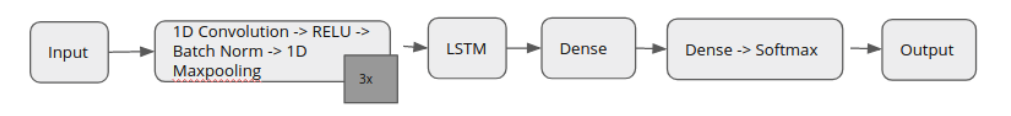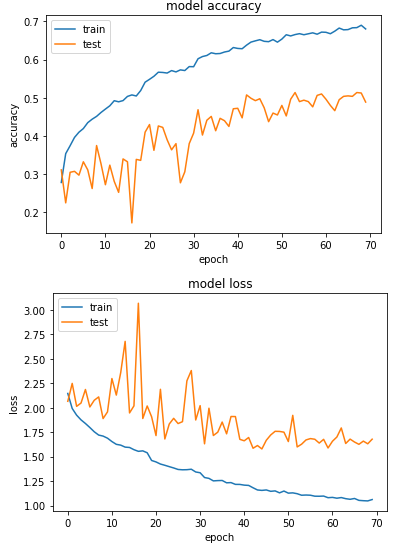Accuracy achieved after 70 epochs of training was 0.48875 . That unfortunately is not much better than baseline models accuracy, but it is a small improvement.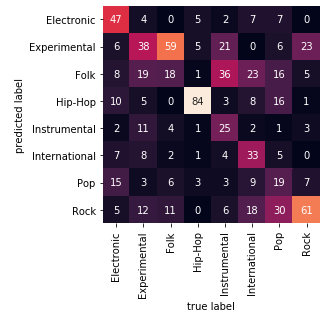From confusion matrix we can see some genres are more likely to be mislabeled as other similar genres. More info and metrics like recall, precision and f1-score, can be found in the notebook with the model itself.

## Confusing the model¶

To confuse the model which is built with the assumption that every track fits exactly one single genre, I tried to pick some tracks which are not easily labeled even if human was to do it.

We move a sliding window of 30s across the track, usually resulting in cca 300-500 30s samples of the given track. We than try to classify each of the samples and plot the results in two different ways.

It was quite storage intensive to process those files, so only resulting csv with the classification results is present in the repository, rest can be downloaded from google drive link found in the readme.

### Absolute ratio plot¶

This plot shows how ratio of detected genres changes over time.

### Sliding window ratio plot¶

This plot looks at last n classification results as time passes and ratio of detected genres is computed for each such window.

### The Prodigy - Voodoo People¶

In :
import pandas as pd
import seaborn as sns
sns.set(rc={'figure.figsize':(11.7,8.27)})
sns.lineplot(data=df,x='time', y='ratio', hue='genre')

Out:
<matplotlib.axes._subplots.AxesSubplot at 0x7f31c09c35c0>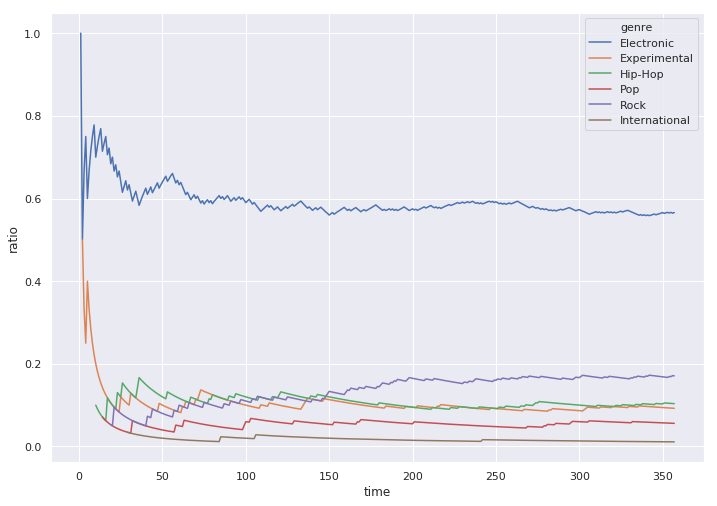In :
df = pd.read_csv("conf_csv/voodoo_people.npz_sliding.csv")
sns.set(rc={'figure.figsize':(11.7,8.27)})
sns.lineplot(data=df,x='time', y='ratio', hue='genre')

Out:
<matplotlib.axes._subplots.AxesSubplot at 0x7f5237642d30>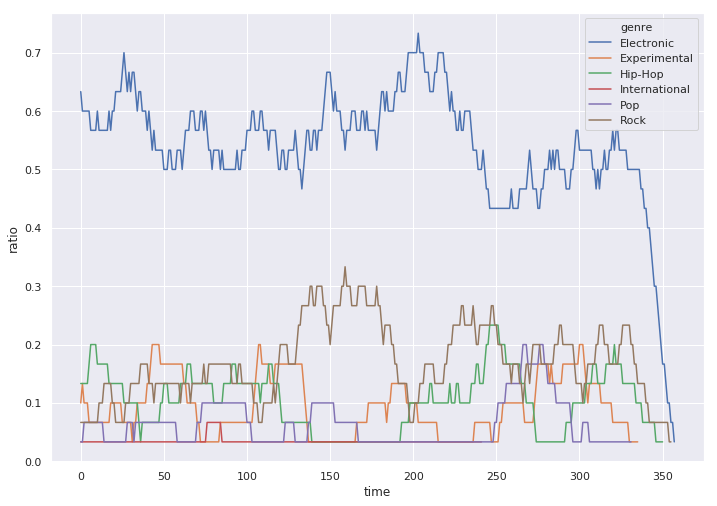### FKY - Ragga 100¶

In :
df = pd.read_csv("conf_csv/ragga100.npz.csv")
sns.set(rc={'figure.figsize':(11.7,8.27)})
sns.lineplot(data=df,x='time', y='ratio', hue='genre')

Out:
<matplotlib.axes._subplots.AxesSubplot at 0x7f5237392438>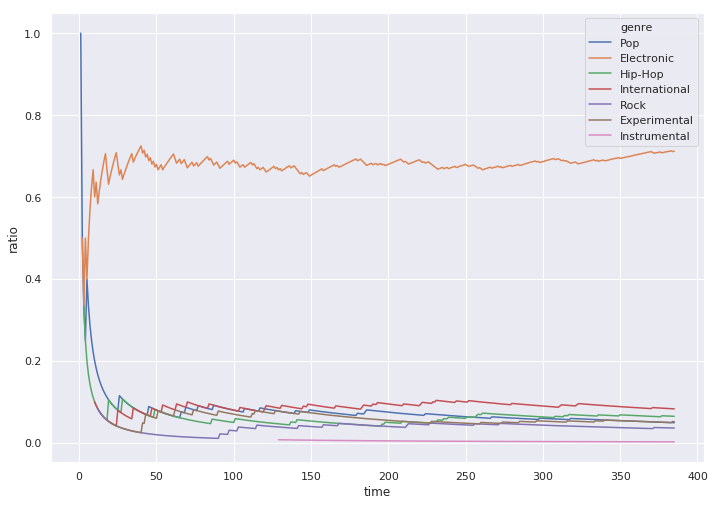In :
df = pd.read_csv("conf_csv/ragga100.npz_sliding.csv")
sns.set(rc={'figure.figsize':(11.7,8.27)})
sns.lineplot(data=df,x='time', y='ratio', hue='genre')

Out:
<matplotlib.axes._subplots.AxesSubplot at 0x7f52374eb860>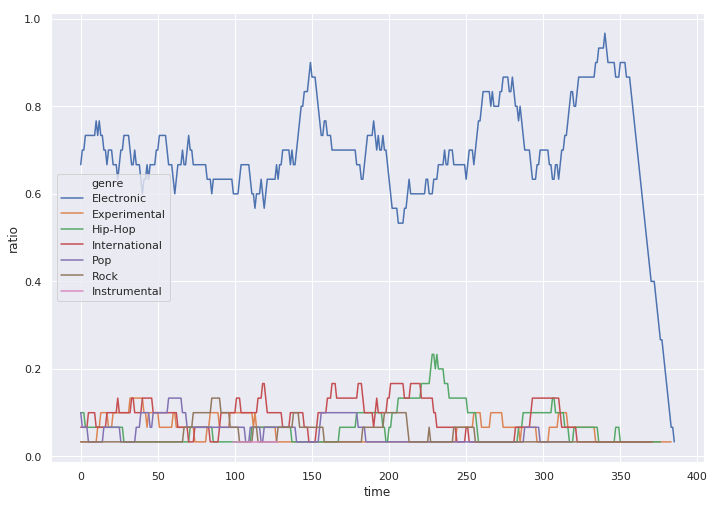### Aphex Twin - Ageispolis¶

In :
df = pd.read_csv("conf_csv/ageispolis.npz.csv")
sns.set(rc={'figure.figsize':(11.7,8.27)})
sns.lineplot(data=df,x='time', y='ratio', hue='genre')

Out:
<matplotlib.axes._subplots.AxesSubplot at 0x7f523749c8d0>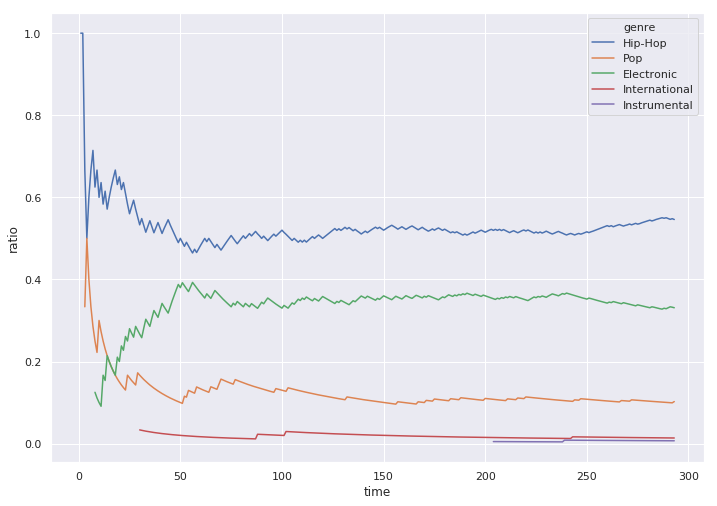In :
df = pd.read_csv("conf_csv/ageispolis.npz_sliding.csv")
sns.set(rc={'figure.figsize':(11.7,8.27)})
sns.lineplot(data=df,x='time', y='ratio', hue='genre')

Out:
<matplotlib.axes._subplots.AxesSubplot at 0x7f52359ef2e8>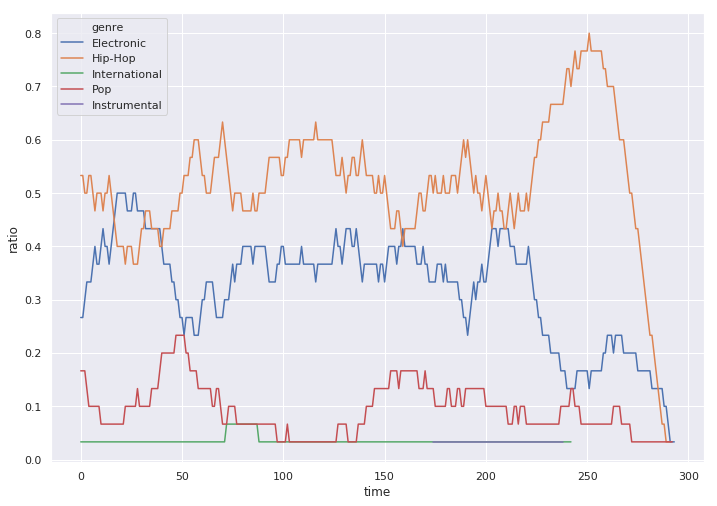### Zone-33 - Sound Of Da Police RMX¶

In :
df = pd.read_csv("conf_csv/police.npz.csv")
sns.set(rc={'figure.figsize':(11.7,8.27)})
sns.lineplot(data=df,x='time', y='ratio', hue='genre')

Out:
<matplotlib.axes._subplots.AxesSubplot at 0x7f5235976048>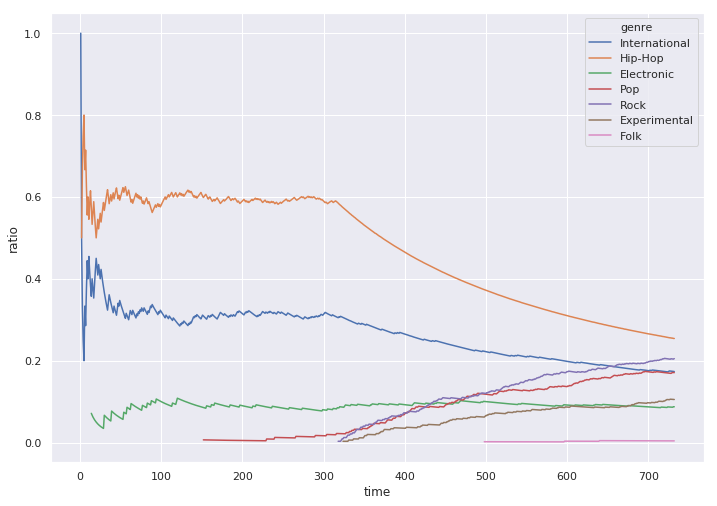In :
df = pd.read_csv("conf_csv/police.npz_sliding.csv")
sns.set(rc={'figure.figsize':(11.7,8.27)})
sns.lineplot(data=df,x='time', y='ratio', hue='genre')

Out:
<matplotlib.axes._subplots.AxesSubplot at 0x7f5235905898>